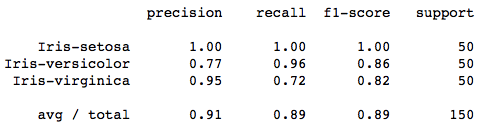# Understanding the Classification report through sklearn

A Classification report is used to measure the quality of predictions from a classification algorithm. How many predictions are True and how many are False. More specifically, True Positives, False Positives, True negatives and False Negatives are used to predict the metrics of a classification report as shown below. The report is copied from our previous post related to K-Means on Iris Dataset.The code to generate a report similar to the one above is:

```from sklearn.metrics import classification_report
target_names = ['Iris-setosa', 'Iris-versicolor', 'Iris-virginica']
print(classification_report(irisdata['Class'],kmeans.labels_,target_names=target_names))```

The report shows the main classification metrics precision, recall and f1-score on a per-class basis. The metrics are calculated by using true and false positives, true and false negatives. Positive and negative in this case are generic names for the predicted classes. There are four ways to check if the predictions are right or wrong:

1. TN / True Negative: when a case was negative and predicted negative
2. TP / True Positive: when a case was positive and predicted positive
3. FN / False Negative: when a case was positive but predicted negative
4. FP / False Positive: when a case was negative but predicted positive

### Precision – What percent of your predictions were correct?

Precision is the ability of a classifier not to label an instance positive that is actually negative. For each class it is defined as the ratio of true positives to the sum of true and false positives.

TP – True Positives
FP – False Positives

Precision – Accuracy of positive predictions.
Precision = TP/(TP + FP)

```from sklearn.metrics import precision_score

print("Precision score: {}".format(precision_score(y_true,y_pred)))```

### Recall – What percent of the positive cases did you catch?

Recall is the ability of a classifier to find all positive instances. For each class it is defined as the ratio of true positives to the sum of true positives and false negatives.

FN – False Negatives

Recall: Fraction of positives that were correctly identified.
Recall = TP/(TP+FN)

```from sklearn.metrics import recall_score

print("Recall score: {}".format(recall_score(y_true,y_pred)))```

### F1 score – What percent of positive predictions were correct?

The F1 score is a weighted harmonic mean of precision and recall such that the best score is 1.0 and the worst is 0.0. Generally speaking, F1 scores are lower than accuracy measures as they embed precision and recall into their computation. As a rule of thumb, the weighted average of F1 should be used to compare classifier models, not global accuracy.

F1 Score = 2*(Recall * Precision) / (Recall + Precision)

```from sklearn.metrics import f1_score

print("F1 Score: {}".format(f1_score(y_true,y_pred)))```

References:

https://scikit-learn.org/stable/modules/generated/sklearn.metrics.classification_report.html

Article Rating
Subscribe
Notify ofInline Feedbacks[…] Understanding the Classification Report […]Ranmal Dewage
7 months ago

Well explained. Keep up the great work!!!

2
0